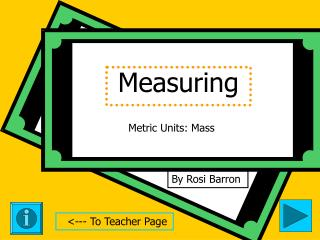DownloadDownload PresentationMeasuring

# Measuring

Télécharger la présentation## Measuring

- - - - - - - - - - - - - - - - - - - - - - - - - - - E N D - - - - - - - - - - - - - - - - - - - - - - - - - - -
##### Presentation Transcript

1. Measuring Metric Units: Mass By Rosi Barron <--- To Teacher Page

2. Fifth Grade Measuring: Metric Measuring Mass • Tips: -Teachers, please read through the whole presentation prior to the activity. -Each activity is meant to be used in addition to your classroom teaching. -Each activity includes a journal component. • Objectives: TSW determine the appropriate metric unit for measuring mass. Teacher Page

3. Units of Mass 1,000 milligrams (mg) = 1 gram (g) 1,000 grams = 1 kilogram (kg) The mass of a paper clip is about 1 gram. Journal each time you see this notepad. ------> Go to activity ------->

4. Choose the more reasonable answer. Explain your answer. --------> A. 30 mg B. 30 g A. 15 g B. 15 kg A. 10 g B. 10 kg A. 1 mg B. 1 kg A. 200 g B. 200 kg A. 4 mg B. 4 kg Did you choose the more reasonable answers? Click here to find out --->

5. These are the more reasonable answers. A. 30 mg B. 30 g A. 15 g B. 15 kg A. 10 g B. 10 kg A. 1 mg B. 1 kg A. 200 g B. 200 kg A. 4 mg B. 4 g GREAT JOB!